Examples

Chapter 8 Class 11 Binomial Theorem
Serial order wise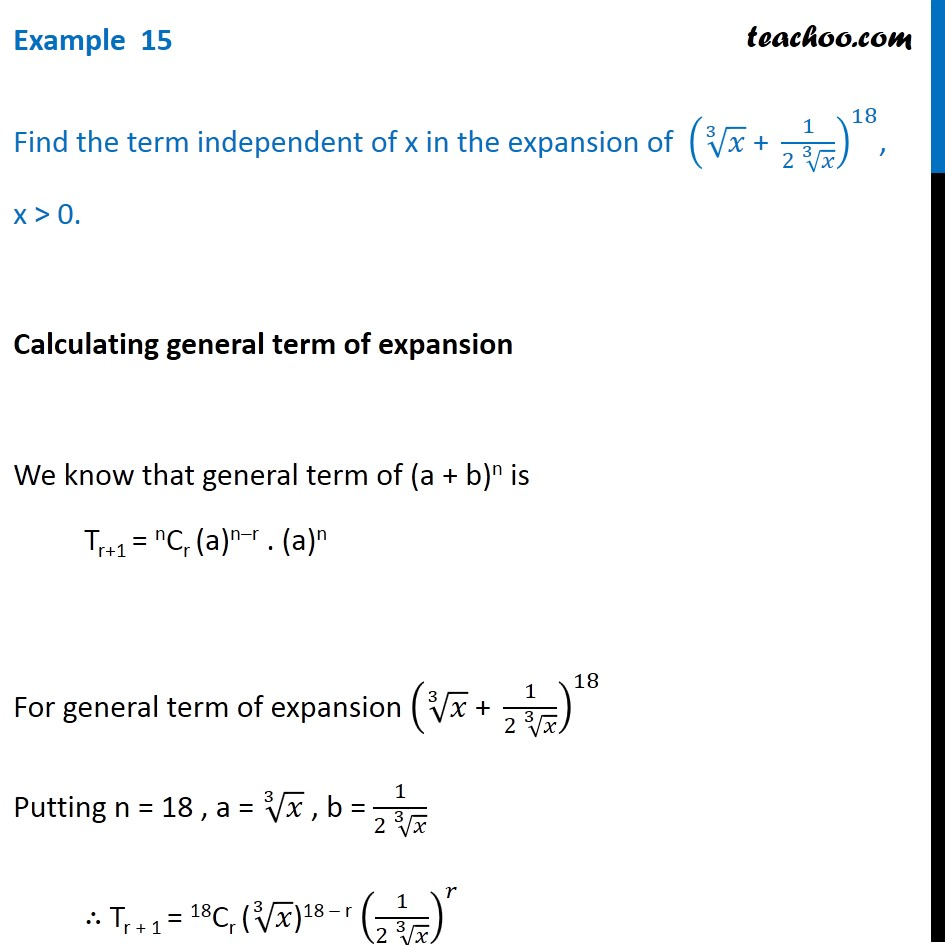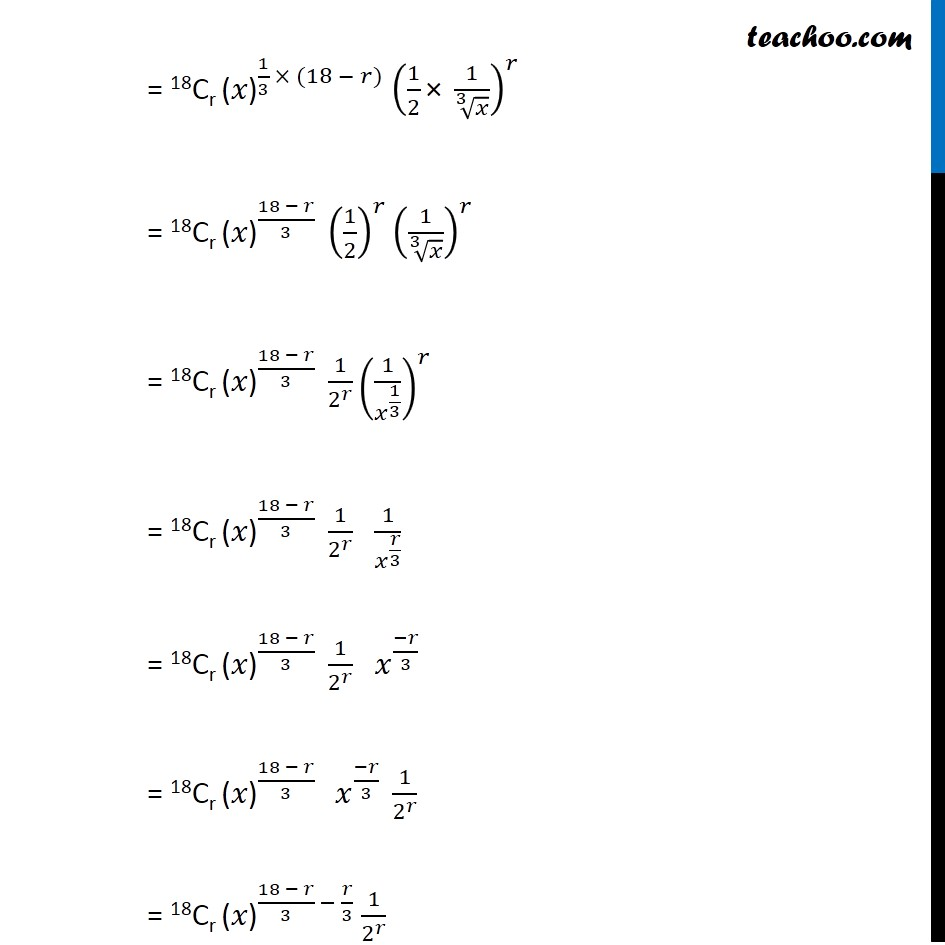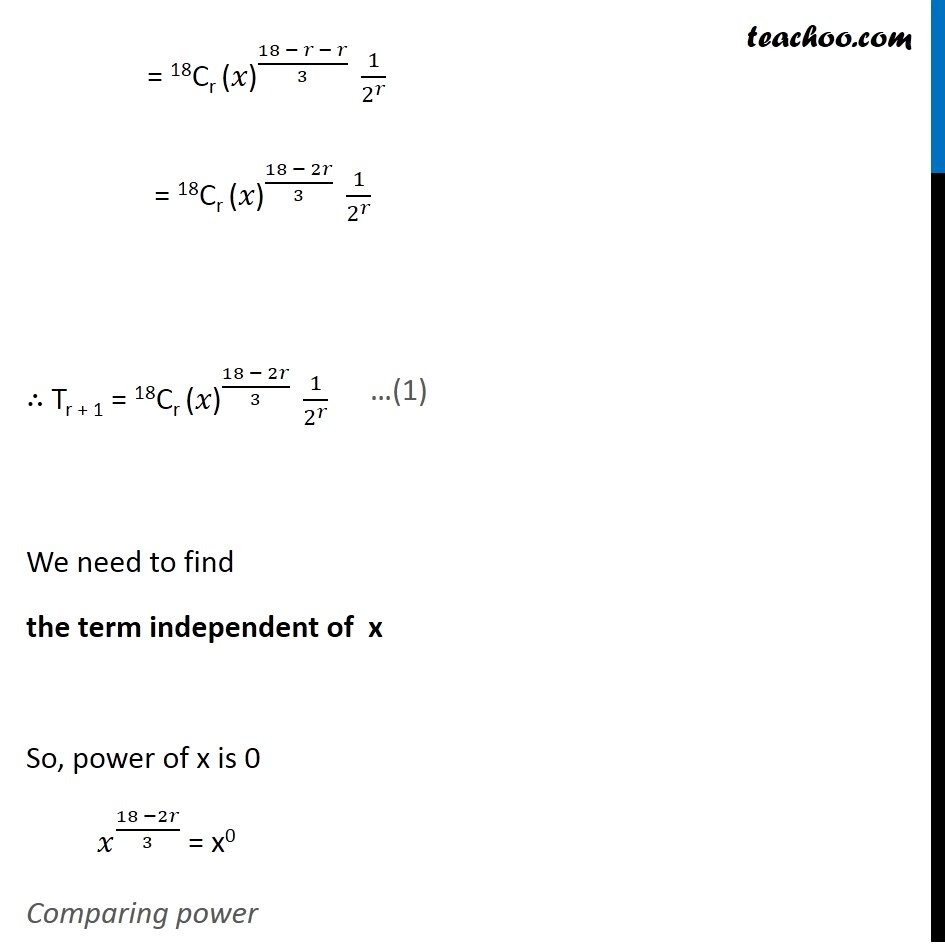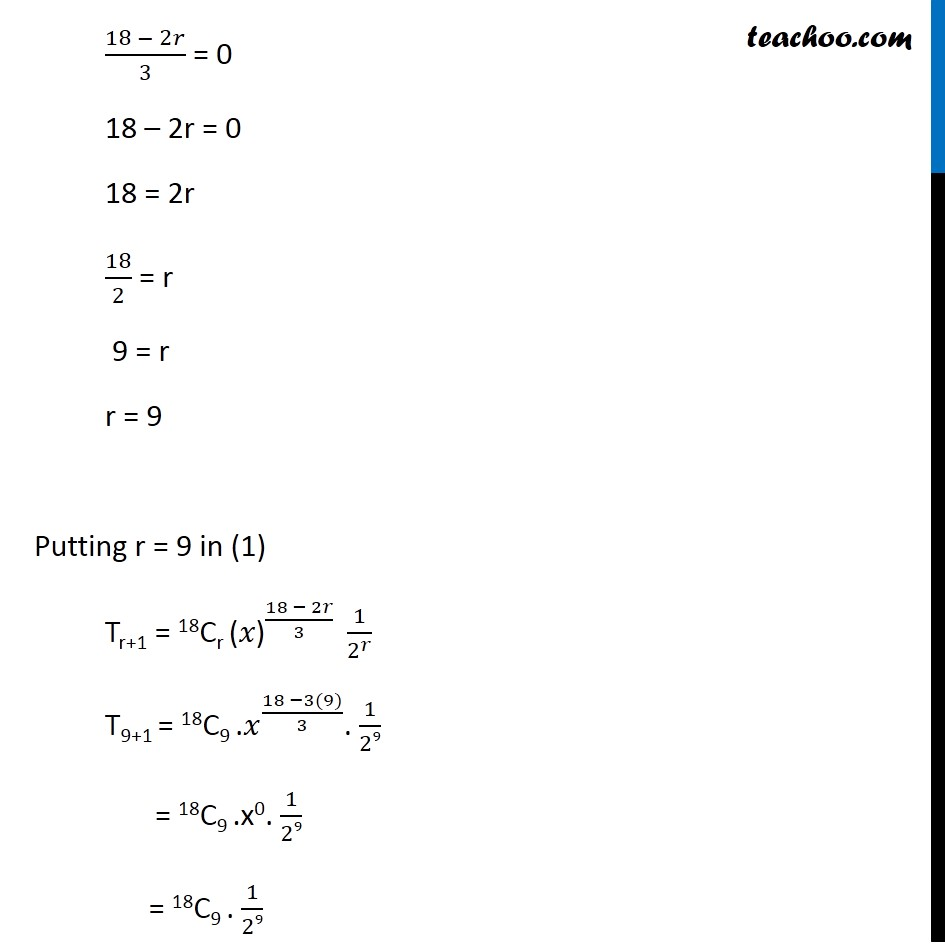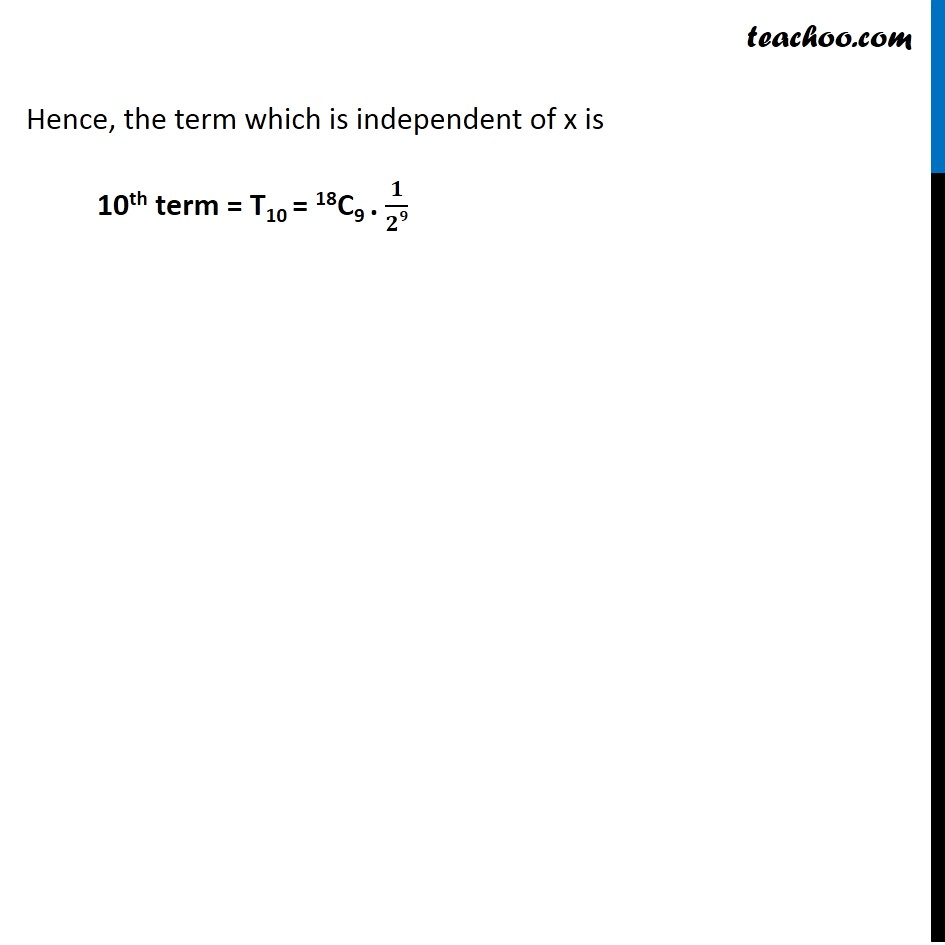Get live Maths 1-on-1 Classs - Class 6 to 12

### Transcript

Example 15 Find the term independent of x in the expansion of (∛𝑥 " + " 1/(2 ∛𝑥))^18, x > 0. Calculating general term of expansion We know that general term of (a + b)n is Tr+1 = nCr (a)n–r . (a)n For general term of expansion (∛𝑥 " + " 1/(2 ∛𝑥))^18 Putting n = 18 , a = ∛𝑥 , b = 1/(2 ∛𝑥) ∴ Tr + 1 = 18Cr (∛𝑥)18 – r (1/(2 ∛𝑥))^𝑟 = 18Cr (〖𝑥")" 〗^(1/3 × (18 − 𝑟)) (1/2 "×" 1/∛𝑥)^𝑟 = 18Cr (〖𝑥")" 〗^((18 − 𝑟)/3 ) (1/2)^𝑟 (1/∛𝑥)^𝑟 = 18Cr (〖𝑥")" 〗^((18 − 𝑟)/3 ) 1/2^𝑟 (1/𝑥^(1/3) )^𝑟 = 18Cr (〖𝑥")" 〗^((18 − 𝑟)/3 ) 1/2^𝑟 1/𝑥^(𝑟/3) = 18Cr (〖𝑥")" 〗^((18 − 𝑟)/3 ) 1/2^𝑟 𝑥^((−𝑟)/3) = 18Cr (〖𝑥")" 〗^((18 − 𝑟)/3 ) 𝑥^((−𝑟)/3) 1/2^𝑟 = 18Cr (〖𝑥")" 〗^((18 − 𝑟)/3 − 𝑟/3) 1/2^𝑟 = 18Cr (〖𝑥")" 〗^((18 − 𝑟 − 𝑟)/3 ) 1/2^𝑟 = 18Cr (〖𝑥")" 〗^((18 − 2𝑟)/3 ) 1/2^𝑟 ∴ Tr + 1 = 18Cr (〖𝑥")" 〗^((18 − 2𝑟)/3 ) 1/2^𝑟 We need to find the term independent of x So, power of x is 0 𝑥^((18 −2𝑟)/3) = x0 Comparing power (18 − 2𝑟)/3 = 0 18 – 2r = 0 18 = 2r 18/2 = r 9 = r r = 9 Putting r = 9 in (1) Tr+1 = 18Cr (〖𝑥")" 〗^((18 − 2𝑟)/3 ) 1/2^𝑟 T9+1 = 18C9 .𝑥^((18 −3(9))/3). 1/29 = 18C9 .x0. 1/29 = 18C9 . 1/29 Hence, the term which is independent of x is 10th term = T10 = 18C9 . 𝟏/𝟐𝟗a